Courses

# Kinetic Theory Of Gases MSQ

## 10 Questions MCQ Test Topic wise Tests for IIT JAM Physics | Kinetic Theory Of Gases MSQ

Description
This mock test of Kinetic Theory Of Gases MSQ for Physics helps you for every Physics entrance exam. This contains 10 Multiple Choice Questions for Physics Kinetic Theory Of Gases MSQ (mcq) to study with solutions a complete question bank. The solved questions answers in this Kinetic Theory Of Gases MSQ quiz give you a good mix of easy questions and tough questions. Physics students definitely take this Kinetic Theory Of Gases MSQ exercise for a better result in the exam. You can find other Kinetic Theory Of Gases MSQ extra questions, long questions & short questions for Physics on EduRev as well by searching above.
*Multiple options can be correct
QUESTION: 1

### A gas in container A is in thermal equilibrium with another gas in container B, both contain equal masses of two gases in the respective containers which of the following can be true? Select one or more:

Solution:

According to the problem, mass of gases are equal so number of moles will not be equal i.e.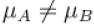From ideal gas equations :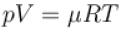∴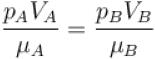(∴ temperature of container are equal)
From this relation, it is clear that if pA = pB then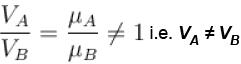Similarly if VA = VB then  i.e. pA ≠ pB.
The correct answers are: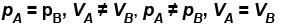*Multiple options can be correct
QUESTION: 2

### Let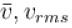and vmp respectively denote mean speed, root mean square speed and most probable speed of the molecules in an ideal monoatomic gas at absolute temperature T. The mass of molecule is m. Then, Select one or more:

Solution:

We know that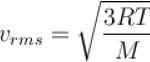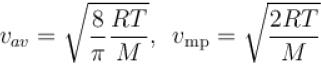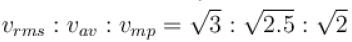so,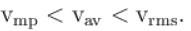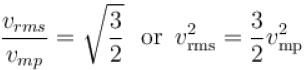∴  Average kinetic energy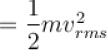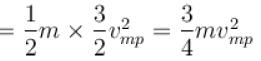The correct answers are: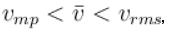The average kinetic energy of a molecule is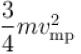*Multiple options can be correct
QUESTION: 3

### Under which of the following conditions is the law pV = RT obeyed most closely by a real gas Select one or more:

Solution:

At low pressure and high temperature real gas obey pV = RT i.e. they behave as ideal gas because at high temperature we can assume that there is no force of attraction or repulsion works among the molecules and the volume occupied by the molecules is negligible in comparison to the volume occupied by the gas.
The correct answers are: Low pressure, High temperature

*Multiple options can be correct
QUESTION: 4

A gas mixture consists of molecules of type 1, 2 and 3 with molar masses m1 > m2 > m3 . vrms and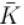are the rms speed and average kinetic energy of the gases. Which of the following are true?
Select one or more:

Solution:

The rms speed depends upon the molecular mass.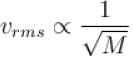but kinetic energy does not depend on it.
i.e.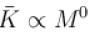On the problem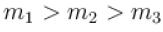∴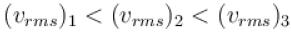But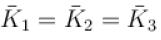The correct answers are: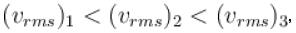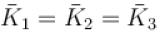*Multiple options can be correct
QUESTION: 5

Which of the following statements are true with regards to mean free path?
Select one or more:

Solution:

As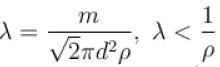i.e. mean free path is inversely proportional to density of a gas. As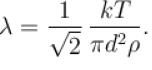For constant volume and thus constant number density n of gas molecule. P/T is constant so, λ will not depend on p and T.
But if volume of given mass of a gas is allowed to change with p and T, then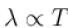at constant pressure and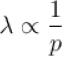at constant temperature.
The correct answers are: Mean free path is inversely proportional to density of gas, For constant volume,  will not depend on T and p

*Multiple options can be correct
QUESTION: 6

Read the given statements and choose which is/are incorrect on the basis of kinetic theory of gases.
Select one or more:

Solution:

If the gas is not ideal then its molecule will posses potential energy. Hence statement (a) is wrong. rms speed of different gases at some temperature depends on its molecules weight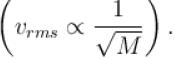Hence, statement (b) is wrong.
Kinetic energy of one gram gas depends on the molecular weight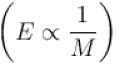. Hence, statement (c) is wrong.
But kinetic energy of one mole of ideal gas does not depend on the molecular weight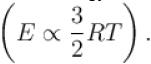Hence (d) is correct.
The correct answers are: Energy of one molecule at absolute to temperature is zero, rms speeds of different gas are same at same temperature, For one gram of all ideal gas, Kinetic energy is same at same temperature.

*Multiple options can be correct
QUESTION: 7

The value of CP – CV = R  for a gas in state A and CP – CV = 1.06R in another state B. If pA and pB denote the pressure and TA and TB denote the temperature in the two state, then
Select one or more:

Solution:

For state A, Cp - CV = R i.e. gas behaves as ideal gas. For state B, Cp – Cv = 1.06R ≠ R i.e. gas does not behave like ideal gas, and we know that at high temperature and at low pressure nature of gas may be ideal so we can say that pA < pB and TA > TB .
The correct answers are: pA < pB , TA > TB

*Multiple options can be correct
QUESTION: 8

Two identical containers each of volume V0 are joined by a small pipe. The containers contains identical gases at temperature T0 and pressure p0 . One container is heated to temperature 2T0 while maintaining the other at same temperature. The common pressure of gas is p and n is the number of the moles of gas in container at temperature 2T0 .
Select one or more:

Solution:

Initially for container A,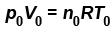For container B, p0 V0 = n0 RT0
∴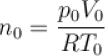Total number of moles =  n0 + n0 = 2n0
Since even on heating, total number of moles is conserved, hence
n1 + n2 = 2n0 .....(1)
If p be the common pressure, then for container A,
pV0 = n1 R2T0
⇒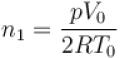For container B,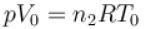⇒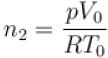Substituting the value of n0 , n1 and n2 is equation (1), we get
⇒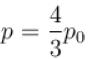Number of mole in container A (at temperature 2T0 )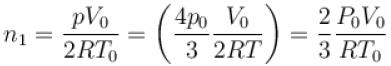The correct answers are: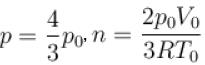*Multiple options can be correct
QUESTION: 9

The equation of state of a gas is given by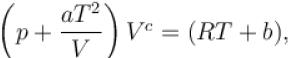where a, b, c, and R are constants. The isotherms can be represented by p = AVm – BVn where A and B depends only on temperature then.
Select one or more:

Solution: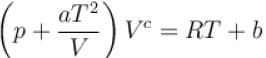⇒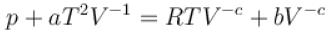⇒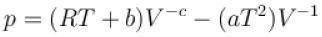Compairing thus with    p = AVm – BVn
we get m = –c and n = –1.
m = -c,
n = –1

*Multiple options can be correct
QUESTION: 10

Which of the following is/are false?
Select one or more:

Solution:

At absolute temperature, kinetic energy of gas molecules becomes zero but they possess potential energy so we can say that at absolute zero degree temperature is not zero energy temperature.
The correct answers are: Two different gases at the same temperature and pressure have equal root mean square velocities, The rms speed of the molecules of different ideal gases, maintained at some temperature are the same, Given sample of ice of hydrogen and ice of oxygen both at NTP oxygen sample has a large number of molecules